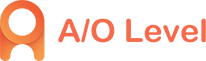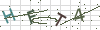• 4008506500
• 1930985500
• peixunmm1
• Suite 601, Level 6, 109 Pitt Street, Sydney,NSW 2000

# A-level数学S1部分的知识点分享

2020-09-28 15:33:35 1432人已读 分享到

在A-level所有科目中，同学们在选择考试科目的时候也有所偏重，A-level数学是很多理科生普遍会选择的科目之一，那么备考A-level数学考试有什么技巧吗？下面就为大家分享S1部分的知识点！

数据的中心及位置测量知识点：

After completing this chapter you should be able to

1.Recognise different types of data

数据的分类

2.Find the mean, median, quartiles and mode for a data set

平均值，中位数,四分位数和众数

3.Find range, interquartile range, variance and standard deviation for a data set

全距，四分位距，方差和标准差

4.Find mean and variance for a combining set of data

找出几组数据之和的平均值和方差

5.Construct and interpret diagrams to represent data—stem and leaf diagram, box-and-whisker plots, histogram, and cumulative frequency graphs

考试主要题型

1.找出几组数据的之和的平均值和方差

2.根据频数分布表画出直方图

3.根据已知数据画出茎叶图，盒式图等

4.数据的图解表示及分析，以及根据图解写出平均值，方差，中位数等;

5.比较两组数据的分布比较

A-Level数学S1知识点1：Recognise different types of data

There are two types of data: qualitative(定性变量)and quantitative(定量变量).

There are two types of quantitative variables, discrete variable and continuous variable.

离散型随机变量可以用频数表表示Frequency distribution for discrete data

连续型随机变量用频数表表示：Frequency distribution for continuous data

To form a frequency distribution of the continuous data, group the information into classes or intervals. When the data is presented as a grouped frequency table, the specific data values are lost.

A-Level数学S1知识点2：Find the mean, median, quartiles and mode for a data set

A-Level数学S1知识点3：Find range, interquartile range, variance and standard deviation for a data set

1.Range全距：highest value-lowest value

2.Interquartile range四分位距：Q3-Q1

3.Variance and standard deviation方差和标准差

Standard deviation gives a measure of the spread of the data in relation to the mean, of the distribution; it is calculated using all the values in the distributions.

For each value x, calculate how far it is from the mean by finding(x-mean).A-Level数学S1知识点4：Find mean and variance for a combining set of data

1.In general, for two sets of data, x and y,

2.In general, if each data value is increased by a constant a

The mean is increased by a

The standard deviation in unaltered.

This is particular useful when finding the mean and standard deviation using and , where a is a constant.

3.Finding the mean and standard deviation using and

a.To find the mean,

Find the mean of (x-a)

b.To find the standard deviation,

Find the standard deviation of (x-a)

This is the same as the standard deviation of x.

A-Level数学S1知识点5：Construct and interpret diagrams to represent data—stem and leaf diagram, box-and-whisker plots, histogram, and cumulative frequency graphs

1.Stem and leaf diagram茎叶图

A way of grouping data into intervals while still retaining the original data is to draw a stem-and-leaf diagram, also known as a stemplot.

These are the marks of 20 students in an assignment:

84 17 38 45 47 53 76 54 75 32

66 65 55 54 51 44 39 19 54 72

In stem-and-leaf diagram all the intervals must be of equal width, so it seems sensible to choose intervals 10-19, 20-29, 30-39,…,80-89 for this data, so tale the stem to represent the tens and the leaf to represent the units.

You enter the numbers one by one. When all the numbers have been entered, you must arrange the entries in each row in numerical order with the smallest nest to the stem.

Important: You must always give a Key to explain what the stem and leaf represent.

Stem-and-leaf diagrams can be used to compare two sets of data by showing them together on a back-to-back stem-and-leaf diagram.

2.Histogram

Grouped data can be displayed in a histogram.

Histograms resemble bar charts, but there are two important differences:

There are no gaps between the bars.

The area of the bar is proportional to the frequency that it represents.

If the height of the bar is adjusted so that the area is equal to the frequency in that interval, then

So , this is known as the frequency density.

3.Box-and-whisker plots盒式图

In a box-and-whisker plot the median and quartiles are shown, as well as the minimum and maximum values of the distribution. It gives a very good visual summary of a distribution and is particularly useful when comparing set of data.

4.Cumulative frequency graphs

Cumulative frequency is the total frequency up to a particular item. Cumulative frequency is particularly useful when finding the median and quartiles.

Cumulative frequency table can be illustrated on a cumulative frequency graph in which the cumulative frequencies are plotted against the upper class boundaries. The points are joined either with a curve or with straight lines.

Values can be estimated from the graph, for example:

(a)Estimate how many plants had a height less than 10.5 cm.

From 10.5 on the x-axis draw a vertical line up to the curve.

Now draw a horizontal line to the y-axis and read off the value

(b)10% of plants had a height of at least x cm. estimate x.

10% of 30=3

3plants had height of at least x cm, so 27 plants had a height less than x cm.

From 27 on the y-axis draw a horizontal line to the curve.

Now draw a vertical line down to the x-axis and read off the value.

From the graph, 10% of the plants had a height of at least 16.5.

以上就是ALevel数学S1知识点及题型分享，希望大家根据自身情况制定合理的复习计划，同时小编提醒大家，在备考中千万不能忽略语法和词汇的重要性！如果想要了解更多关于如何学习ALevel、ALevel自学考试的信息，可以在线咨询我们或添加客服微信kewo11！• ### alevel考试备考词汇积累（二十六）4008506500